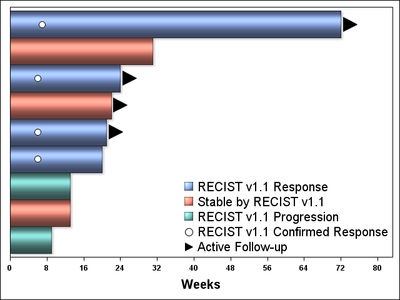## need larger arrowheads in my swim lane plot

Hi,

I'm working on the attached swim lane plot using the code also attached.  The anno2 dataset in the code creates the current arrowheads using the "arrow" function, but it doesn't have any options to increase the size.  I would like the "ongoing" arrowheads at the end of the time on study bars to be larger, but haven't found any way to do that.  I'm using SAS 9.4 and would appreciate any help with this issue.

Swannie

1 ACCEPTED SOLUTION

Accepted SolutionsJay54
Meteorite | Level 14

## Re: need larger arrowheads in my swim lane plot

You really do not need any annotation at all.  See attached code and graph.

Just like you used scatter plot to render the circle, you can do the same for the arrowhead.

The legend is automatically built for you.  You can easily reorder the entries if needed.``````proc format;
value recist
1='RECIST v1.1 Response'
2='Stable by RECIST v1.1'
3='RECIST v1.1 Progression';
run;

data tos;
set data.swim_lane;
if ongoing='y' then loc=tos+2;
run;

ods graphics / reset imagename='Swim_Lane_2';
proc sgplot data=tos ;
format group recist.;
scatter X=ttrc Y=subjn /markerattrs=(symbol=circlefilled size=12 color=black) filledoutlinedmarkers
markerfillattrs=(color=white) markeroutlineattrs=(color=black) legendlabel='RECIST v1.1 Confirmed Response';
scatter y=subjn x=loc / markerattrs=(symbol=trianglerightfilled size=24) legendlabel='Active Follow-up';
keylegend / location=inside position=bottomright noborder across=1 valueattrs=(size=14) autoitemsize;
yaxis type=discrete display=(noline novalues noticks nolabel) labelattrs=(size=18 weight=bold);
xaxis type=linear labelattrs=(size=14 weight=bold) VALUEATTRS= (Weight=Bold) label="Weeks" values=(0 to 80 by 8) offsetmin=0;
run;``````

7 REPLIES 7Jay54
Meteorite | Level 14

## Re: need larger arrowheads in my swim lane plot

If you are using SGPLOT, you have many ways to do the arrow.  You can use the HighLow plot that has a built in arrowhead.  Or overlay a SCATTER with the arrow type symbols.  You can adjust the size as you want.

http://blogs.sas.com/content/graphicallyspeaking/2014/06/22/swimmer-plot/

## Re: need larger arrowheads in my swim lane plot

Thanks for the quick response, but is there some way to do this that doesn't involve reprogramming the dataset and production code?  I'd prefer not to have to go back and do that at this point.

SwannieJay54
Meteorite | Level 14

## Re: need larger arrowheads in my swim lane plot

Please attach your code with some sample data so we can run it to see what you are doing.  What release of SAS (including maintenance level) are you using?

## Re: need larger arrowheads in my swim lane plot

Hmmm.  I tried to attach both the figure and the code in my first post.  Is there some trick to attaching more than one file?  Seems like there is, because I can only get one at a time to work.  I put the files in a .zip file; hoping that works.

Swannie

## Re: need larger arrowheads in my swim lane plot

What code are you using?  I would guess either style or proc template update.  You may even find an example at:

http://blogs.sas.com/content/graphicallyspeaking/Jay54
Meteorite | Level 14

## Re: need larger arrowheads in my swim lane plot

You really do not need any annotation at all.  See attached code and graph.

Just like you used scatter plot to render the circle, you can do the same for the arrowhead.

The legend is automatically built for you.  You can easily reorder the entries if needed.``````proc format;
value recist
1='RECIST v1.1 Response'
2='Stable by RECIST v1.1'
3='RECIST v1.1 Progression';
run;

data tos;
set data.swim_lane;
if ongoing='y' then loc=tos+2;
run;

ods graphics / reset imagename='Swim_Lane_2';
proc sgplot data=tos ;
format group recist.;
scatter X=ttrc Y=subjn /markerattrs=(symbol=circlefilled size=12 color=black) filledoutlinedmarkers
markerfillattrs=(color=white) markeroutlineattrs=(color=black) legendlabel='RECIST v1.1 Confirmed Response';
scatter y=subjn x=loc / markerattrs=(symbol=trianglerightfilled size=24) legendlabel='Active Follow-up';
keylegend / location=inside position=bottomright noborder across=1 valueattrs=(size=14) autoitemsize;
yaxis type=discrete display=(noline novalues noticks nolabel) labelattrs=(size=18 weight=bold);
xaxis type=linear labelattrs=(size=14 weight=bold) VALUEATTRS= (Weight=Bold) label="Weeks" values=(0 to 80 by 8) offsetmin=0;
run;``````

## Re: need larger arrowheads in my swim lane plot

Fantastic!  Very elegant solution.  Thanks very much, Swann

Discussion stats
• 7 replies
• 1141 views
• 0 likes
• 3 in conversation